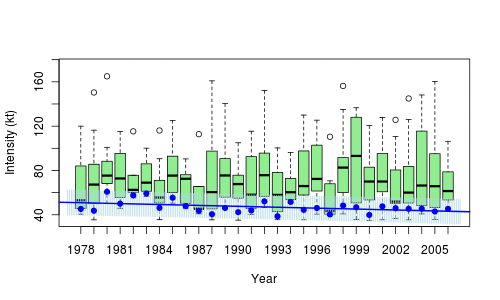# Generating Hurricanes with a Markov Spatial Process

The National Hurricane Center (NHC) collects datasets with all  storms in North Atlantic, the North Atlantic Hurricane Database (HURDAT). For all sorms, we have the location of the storm, every six jours (at midnight, six a.m., noon and six p.m.). Note that we have also the date, the maximal wind speed – on a 6 hour window – and the pressure in the eye of the storm.

It is possible to run the following function

```library(XML)
extract.track=function(year=2012,p=TRUE){```

# Talk on quantiles at the R Montreal groupThis afternoon, I will be giving a two-hour talk at McGill on quantiles, quantile regressions, confidence regions, bagplots and outliers. Before defining (properly) quantile regressions, we will mention regression on (local) quantiles, as on the graph below, on hurricanes,In order to illustrate quantile regression, consider the following natality database,

```base=read.table(
"http://freakonometrics.free.fr/natality2005.txt",

We can use it produce those nice graphs we can find in several papers, modeling weight of newborns,

```u=seq(.05,.95,by=.01)
coefstd=function(u) summary(rq(WEIGHT~SEX+
SMOKER+WEIGHTGAIN+BIRTHRECORD+AGE+ BLACKM+
BLACKF+COLLEGE,data=base,tau=u))\$coefficients[,2]
coefest=function(u) summary(rq(WEIGHT~SEX+
SMOKER+WEIGHTGAIN+BIRTHRECORD+AGE+ BLACKM+
BLACKF+COLLEGE,data=base,tau=u))\$coefficients[,1]
CS=Vectorize(coefstd)(u)
CE=Vectorize(coefest)(u)```The slides can be downloaded on the blog, as well as the R-code.